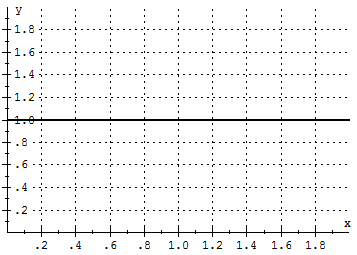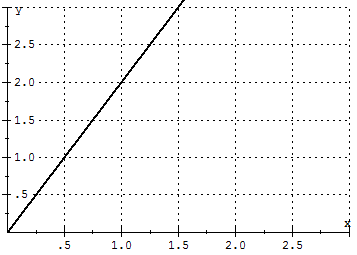# Calculus Problem of the Week October 14, 2011

Sometimes easy and sometimes hard, our calculus problem of the week could come from any calculus topic. If you really want to get better at calculus, following these problems is a great way to make yourself practice! Past calculus problems of the week.

This week’s problem:
(click “see the solution” at the bottom of post to, well, see the solution. If it doesn’t work, please click the title of this post and then try (I’m working on this!))

Continuing in the direction from last week, this week try to find an example of a function$f(x)$ such that the definite integral from 0 to 1 of$f(x)$ is exactly 1. You should be able to think of at least two different “types” of functions if you want to make it more interesting!
See the solution.

Ok, maybe this is pushing it, but what I am IMAGINING you doing and HOPING you are doing is thinking about what an integral actually is. An integral in this sense is a way of measuring AREA. So really, you are trying to think of a function that would have an area of 1 under it from 0 to 1.

One example of such a function is$f(x)=1$ which is graphed below:As you can see, from 0 to 1, the area under the curve is the area of the square with sides of length 1 so the area would be 1. This means the integral will be 1 as well and if you check:$\int_{0}^{1}(1) dx=x|_{0}^{1}=1$

What else? Well, could we get a triangle in that space so that it would have an area of 1? Triangles have area$\dfrac{1}{2}bh$ where b is for base and h is for height. The integral is from 0 to 1 so the base would be 1 – therefore the height would have to be 2 to get an area of 1. Ok, that means it would have to go through the points (0,0) and (1,2) and be a straight line… What function can we get to make that happen?Aha!$f(x)=2x$ will work! Check the integral like we did above to see.